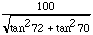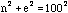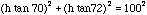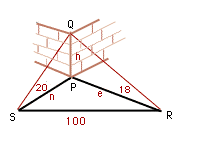Subject: Trigonometric functions
Date: Fri, 19 Dec 1997 03:35:15 PST

My name is Calvin and I have a year 12 question for you to help me with.

From a point S, the angle of elevation of the top of a tower due north of it is 20 degrees. From R, due east of the tower, the angle of elevation is 18 degrees. S and R are 100m apart. Show that the height of the tower is given bythank you very much

Hi Calvin

 In the diagram I have called the height of the building h, the distance from the building to the north measuring point n and the distance from the building to the east measuring point e. Since angle QSP is 20 degrees and angle SPQ is a right angle, the angle PQS is 70 degrees. Likewise angle RQP is 72 degrees. Thus n/h=tan70 and e/h=tan72, ie n=h*tan70 and e=h*tan72. But triangle RPS has a right angle at P soand hence. Solving this equation for h gives the expression required.Cheers
Harley

Go to Math Central

To return to the previous page use your browser's back button.